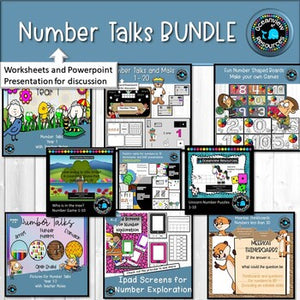Oceanview Education and Teaching Supplies

# Early Math BUNDLE numbers 1-20 worksheets and discovery mats

Regular price \$25.00 \$0.00 Unit price per
Tax included at checkout

Encourage students to discuss their answers with their peers.

How did they reach the answer?

What were they thinking in their head?

What facts did they already know that helped them?

What did they see?

Use the powerpoint to model the different ways that we can visualise a given number. This set comprises the numbers 1-20, and shows the number

• in a ten frame
• written as a word
• using hands
• domino patterns
• dice patterns
• subitising patterns
• clipart version of the number
• tally marks

The powerpoint is editable so that you can change to the text that you require, and then save as a PDF prior to printing.

Ideal for students in their first term of school to establish and consolidate basic number facts.

Represent and solve simple addition and subtraction problems using a range of strategies, including counting

on, partitioning and rearranging parts

uses a range of strategies and informal recording methods for addition and subtraction involving one- and two-digit numbers

Use and record a range of mental strategies to solve addition and subtraction problems involving one- and two-digit numbers, including:

− counting on from the larger number to find the total of two numbers

− counting back from a number to find the number remaining

− counting on or back to find the difference between two numbers

− using doubles and near doubles,

− combining numbers that add to 10

− bridging to 10

− using place value to partition numbers

Choose and apply efficient strategies for addition and subtraction

Represent and solve simple addition and subtraction problems using a range of strategies, including counting

on, partitioning and rearranging parts

quses a range of strategies and informal recording methods for addition and subtraction involving one- and two-digit numbers

Use and record a range of mental strategies to solve addition and subtraction problems involving one- and two-digit numbers, including:

− counting on from the larger number to find the total of two numbers

− counting back from a number to find the number remaining

− counting on or back to find the difference between two numbers

− using doubles and near doubles,

− combining numbers that add to 10

− bridging to 10

− using place value to partition numbers

Choose and apply efficient strategies for addition and subtraction

This is for use by one teacher in one classroom. If you would like to share with your colleagues, PLEASE purchase a multiple license. Thank you :)